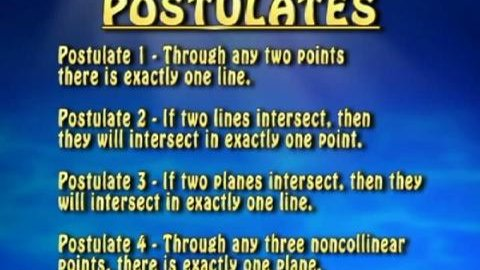# Zero The Math Hero - Geometry Tutor

##### TMW Media## 12 videos in this playlist

Points, Lines & Planes
This program teaches the properties of basic shapes of 0, 1 and 2 dimensions and an explanation of the relationship between points, lines and planes. Four basic postulates are taught…
Segments, Rays & Angles
This program teaches three important geometric concepts and an explanation of the different angle types based on their measures, using units called degrees. Students will Learn: Segments: identify segments, name…
Segment Postulates & Angle Postulates
This program teaches using coordinates on a number line to find segment lengths along with the proper use of a protractor to measure angles. It uses problem solving to find…
Coordinate Plane
This program teaches the coordinate plane, its parts and how it is used to graph points on a 2-dimensional coordinate system. It also offers practical modern-day application of the coordinate…
Classifying Triangles
This program provides a discussion of the basic definition of a triangle and demonstrates how to classify triangles according to their side lengths and/or angle measures. The following Triangle types…
Finding Triangle Measures
This program introduces the concept of a theorem and models how to find angle measures for interior and exterior angles for a variety of triangles. Several examples are demonstrated, using…
This program discusses two important theorems and defines the term polygon as well as specific polygon types. Problem solving is used to find missing measures for both interior and exterior…
Pythagorean Theorem
This program teaches four important theorems and describes the Pythagorean Theorem and its relevance in the real world. Several example problems are illustrated. Problems using algebraic skills are linked with…
Circles & Arcs
This program defines the term circle and how to find angle measures for central angles and arcs. The distance formula is used to find the length of a radius on…
Perimeter, Circumference & Area
This program defines perimeter, circumference and area of 2-dimensional figures. The concept of the number pi is also represented with different approximations and many examples of finding perimeter and area…
Lateral Area & Surface Area
This program describes how to identify the correct parts of the figures so students will be able to substitute them into formulas. The Pythagorean Theorem is also used in the…
Volume & Introduction to Proofs
This program describes how to identify the correct parts of the figures so students will be able to substitute them into the formulas. The Pythagorean Theorem is also used in…

## Related videos

Probability and Statistics Tutor Series
The Probability and Statistics Tutor is a complete 14 lesson series covering all of the core topics in detail. What sets this series apart from other teaching tools is that the concepts are taught entirely through step-by-step example problems of increasing difficulty. It works by introducing each new concept in…
Geometry
Key concepts: formulas for a parallelogram (square, rectangle, and rhombus) & formulas for a trapezoid & triangle.
Math Series 1
A math series designed for grades 7th to 9th to help students learn and understand math concepts.
Math Series 2
Math lessons including Geometry, Probability, Problem Solving, Trigonometry and Ratios.
Algebra: A Piece of Cake
Covers variable, numerical substitution, algebraic conventions, developing algebraic formulas from number patterns.
Factoring is Fantastic
Key concepts explained: Factoring, terms, expressions, finding the greatest common factor(s), and factoring polynomial expressions that are the sums or differences of terms with common factors. This starts with a review of numerical factors and greatest common factors, and moves into the realm of algebra. Different types of factoring and…
Integer Operations into Negative Zone
Key concepts explained: negative numbers, integers, addition and subtraction of integers.
Linear Equations and Their Graphs
Key concepts explained: linear equations and their graphs, and the domain of an equation.
Percentages: That Make Sense
Part of the Series: Math Series 1
Key concepts explained: converting percentages into decimals and fractions, and converting decimals and fractions into percentages. Taking examples from the widespread use of percentages in everyday life from the percentage of cappuchinos to regular coffee a coffee shop sells, to the percentage of sharks to other fish in an aquarium,…
Slopes: That's a Little bit Steep
Part of the Series: Math Series 1
Key concepts explained: horizontal and vertical lines, positive and negative slopes, calculating a slope by measuring its rise and run, and calculating a slope by using two points on a coordinate plane.
Probability
Expressing a probability between certainty and impossible as the likelihood of an uncertain event occurring --conducting and recording probability experiments using small and large number trials with replacement --calculating the theoretical probability of a particular event occurring --expressing probabilities as odds